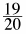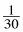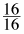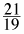### Home > MC1 > Chapter 8 > Lesson 8.2.2 > Problem8-39

8-39.

Describe how each of the following multipliers would change the dimensions of a photograph.

 a.b.c.d.If the fraction is less than one, does it make sense that the photograph would become smaller?
The opposite is true if the fraction is greater than one.
If the fraction is much less than one, the photograph will be significantly reduced.
If it is just a little less than one, it will only be reduced a small amount.

Knowing the information above, can you now describe how the dimensions would change?

With the multiplier in part (b), the photograph would be significantly smaller.
With the multiplier in part (c), the photograph would stay the same size.
Can you tell how the multipliers in parts (a) and (d) would change the photograph?